# Q1) [50 points] Infinitely long wire is placed onto y-axis 7 and right angled trapezoid frame is placed onto xy-plane as in Figure 1. 11 and 12 currents flow into the wire and the frame respectively...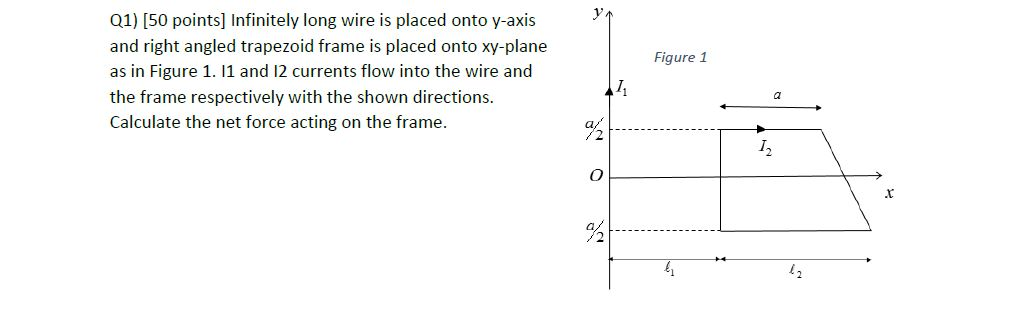Q1) [50 points] Infinitely long wire is placed onto y-axis 7 and right angled trapezoid frame is placed onto xy-plane as in Figure 1. 11 and 12 currents flow into the wire and the frame respectively with the shown directions. Figure 1 Calculate the net force acting on the frame. 2 I. .t 42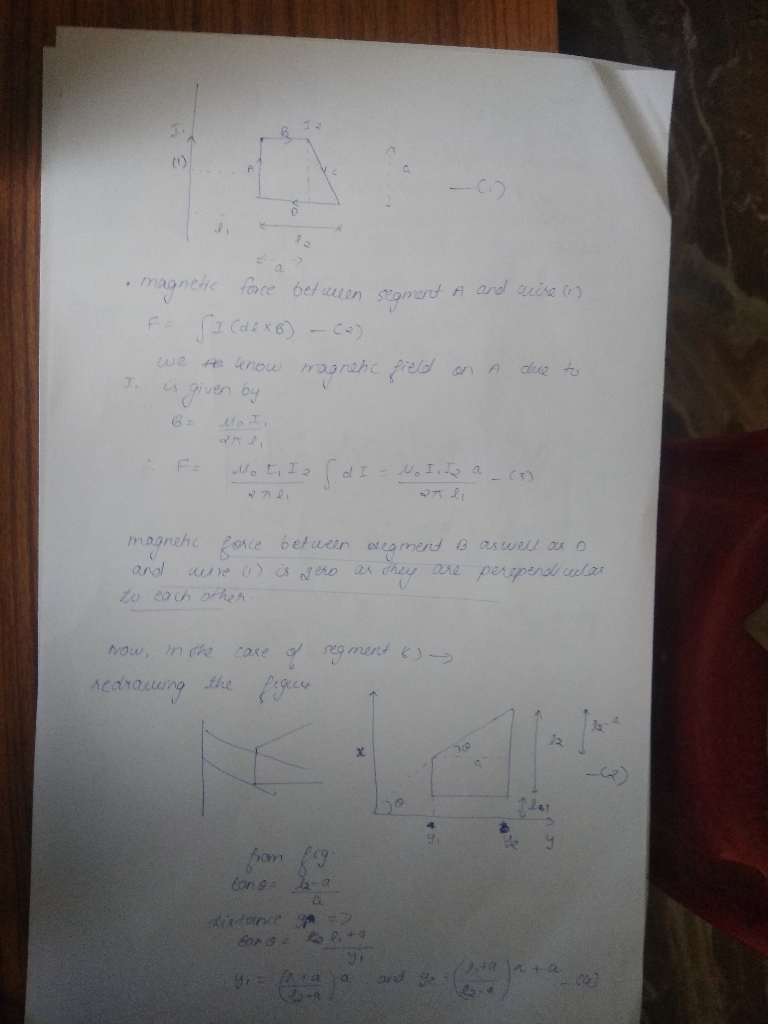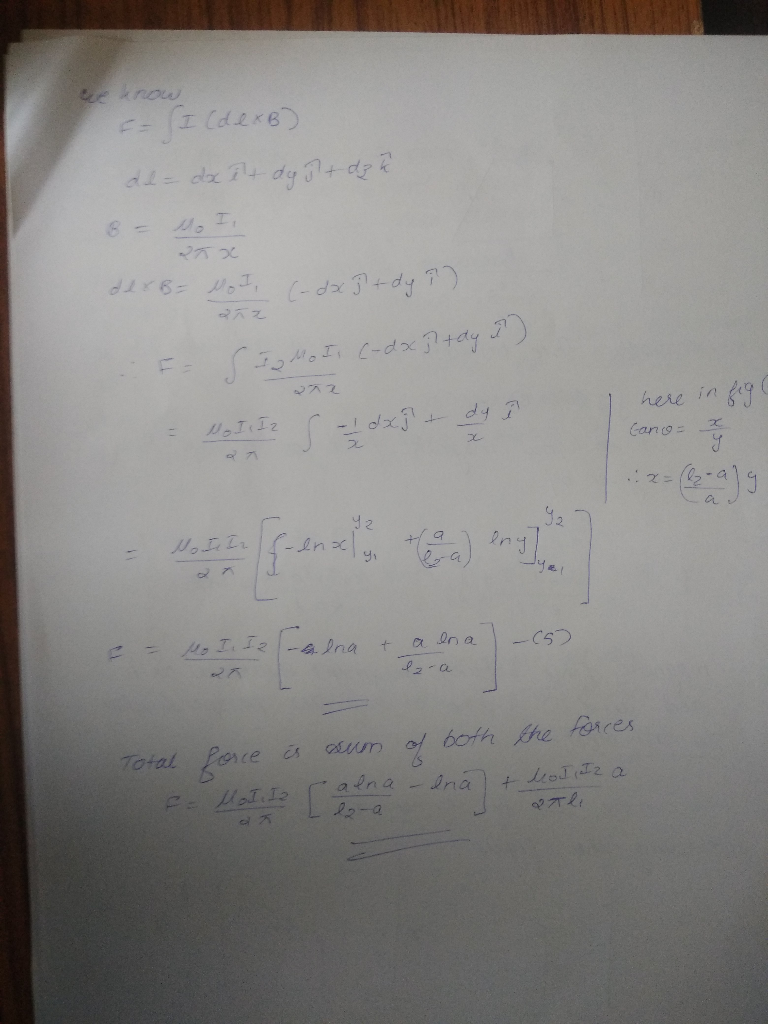##### Add Answer of: Q1) [50 points] Infinitely long wire is placed onto y-axis 7 and right angled trapezoid frame is placed onto xy-plane as in Figure 1. 11 and 12 currents flow into the wire and the frame respectively...
Similar Homework Help Questions
• ### Q1) [50 points] Infinitely long wire is placed onto y-axis and right angled trapezoid frame is...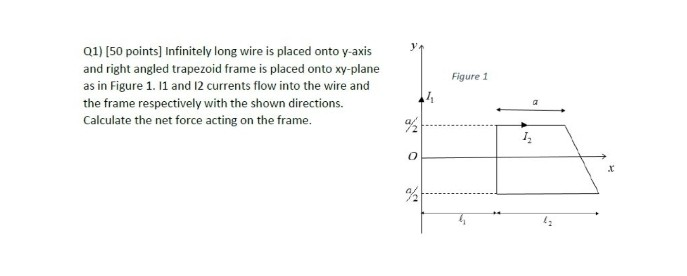Q1) [50 points] Infinitely long wire is placed onto y-axis and right angled trapezoid frame is placed onto xy-plane as in Figure 1. 1 and 12 currents flow into the wire and the frame respectively with the shown directions. Calculate the net force acting on the frame. Figure1 I,

• ### It is a basic electromagnetic fields question. can you help me? Infinitely long wire is placed onto y-axis and right angled trapezoid frame is placed onto xy-plane as in Figure 1. 11 and 12 currents...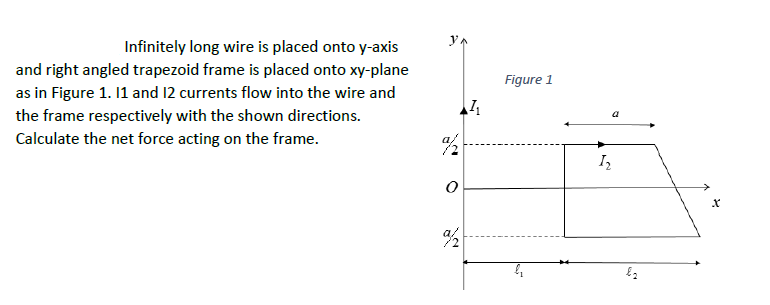It is a basic electromagnetic fields question. can you help me? Infinitely long wire is placed onto y-axis and right angled trapezoid frame is placed onto xy-plane as in Figure 1. 11 and 12 currents flow into the wire and the frame respectively with the shown directions Calculate the net force acting on the frame. Figure 1 a/ /2 Infinitely long wire is placed onto y-axis and right angled trapezoid frame is placed onto xy-plane as in Figure 1. 11...

• ### Wire 1 and wire 2 are in the xy plane and they are parallel to the...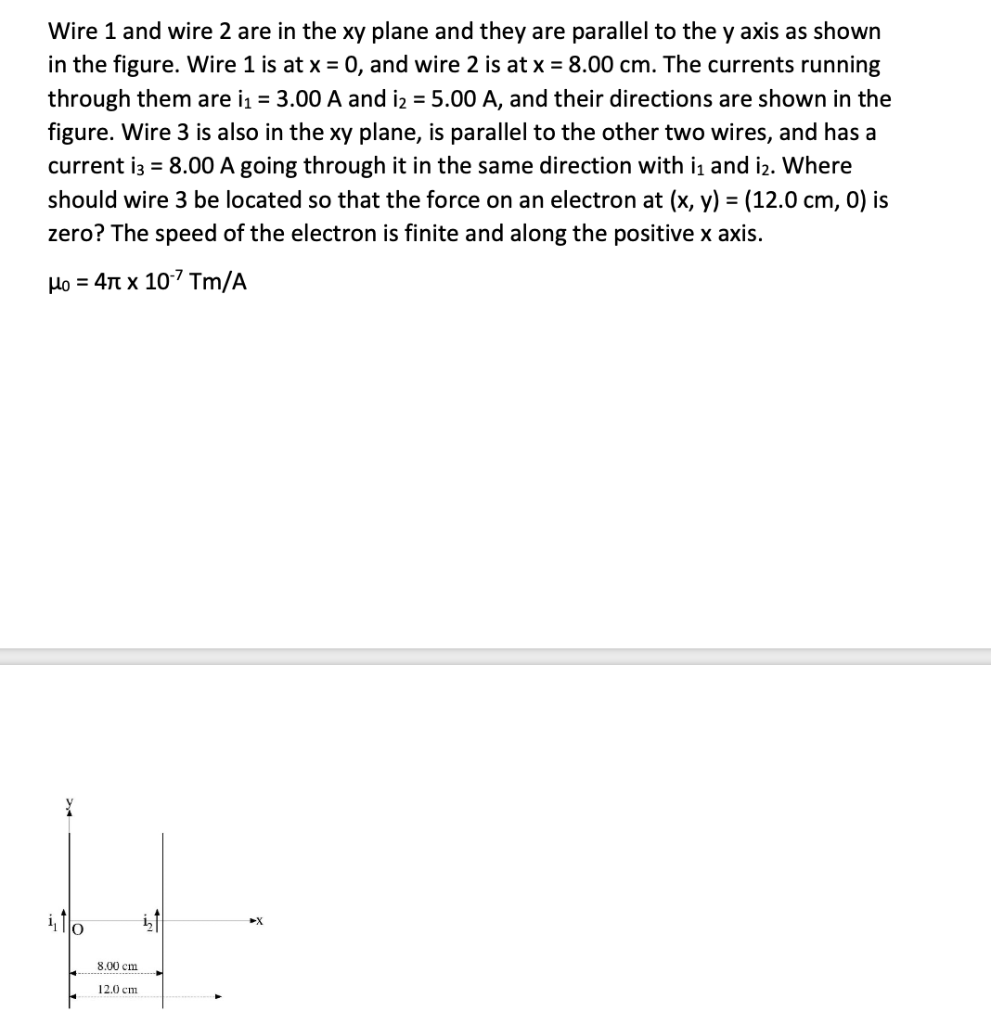Wire 1 and wire 2 are in the xy plane and they are parallel to the y axis as shown in the figure. Wire 1 is at x = 0, and wire 2 is at x = 8.00 cm. The currents running through them are i1 = 3.00 A and 12 = 5.00 A, and their directions are shown in the figure. Wire 3 is also in the xy plane, is parallel to the other two wires, and has a...

• ### Question 20 (7 points) Two long straight wires carry currents perpendicular to the xy-plane. One wire...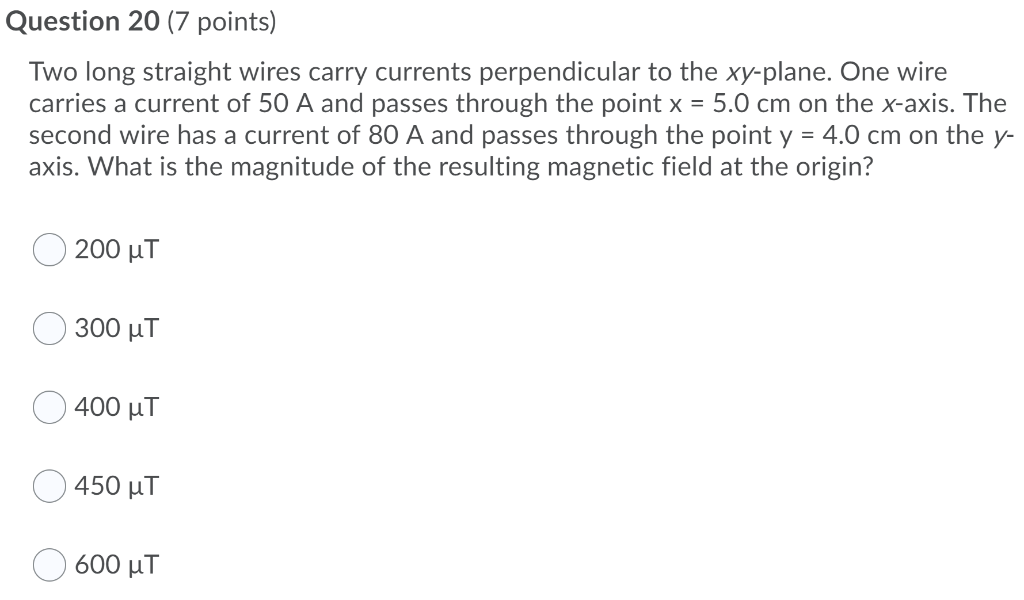Question 20 (7 points) Two long straight wires carry currents perpendicular to the xy-plane. One wire carries a current of 50 A and passes through the point x = 5.0 cm on the x-axis. The second wire has a current of 80 A and passes through the point y = 4.0 cm on the y- axis. What is the magnitude of the resulting magnetic field at the origin? 200 ut 300 uT 400 uT 450 uT 600 uT

• ### Three questions. Thanks 1. One long wire carries current 12.0 A to the left along the...

Three questions. Thanks 1. One long wire carries current 12.0 A to the left along the x axis. A second long wire carries current 80.0 A to the right along the line (y = 0.280 m, z = 0). (a) Where in the plane of the two wires is the total magnetic field equal to zero? (b) A particle with a charge of −2.00 µC is moving with a velocity of 150î Mm/s along the line (y = 0.100 m,...

• ### Point along the wire, the sum of current entering the junction must equal the the current exiting...point along the wire, the sum of current entering the junction must equal the the current exiting that junc tion. This is Kirchhoff current law (KCL). An isolated wire segment supports zero DC current. A current loop shown in Fig. 10 fulfills KCL as the current is continuous throughout the loop. tega歹98g 顛@l The magnetic field of a current loop forms loops along the current loop as depicted in Fig. 10 with direction consistent with the right hand rule. Away...

• ### 0.50 T. In unitve is the torque about the hinge MAGNETIC FIELDS MAG 3 An clectron...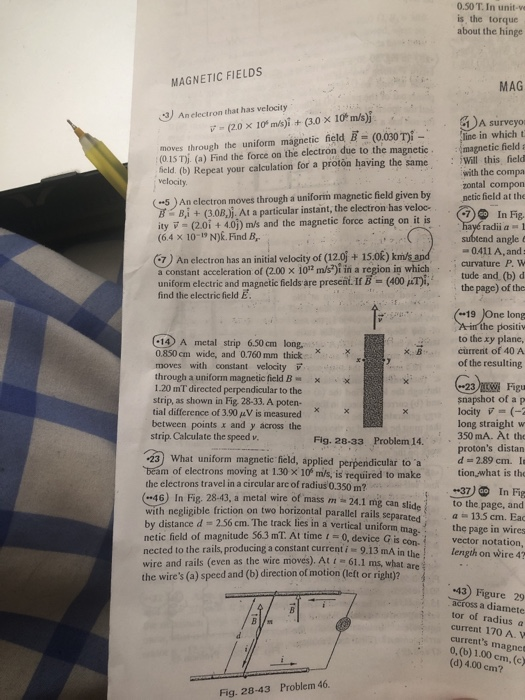0.50 T. In unitve is the torque about the hinge MAGNETIC FIELDS MAG 3 An clectron that has velocity 7- (20 x 10m/s)i + (3.0 x 10 m/s) moves through the uniform magnetic field B = (0.030 Ti - (0.15 T). (a) Find the force on the electron due to the magnetic field. (b) Repeat your calculation for a proton having the same velocity (-5) An electron moves through a uniform magnetic field given by B Bi + (3.08.). At...

Free Homework App